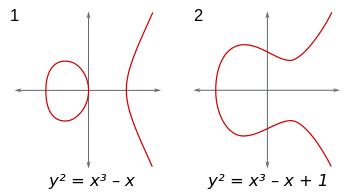# Modular elliptic curveA modular elliptic curve is an elliptic curve E that admits a parametrisation X0(N)  E by a modular curve. This is not the same as a modular curve that happens to be an elliptic curve, and which could be called an elliptic modular curve. The modularity theorem, also known as the Taniyama–Shimura conjecture, asserts that every elliptic curve defined over the rational numbers is modular.

## History and significance

In the 1950s and 1960s a connection between elliptic curves and modular forms was conjectured by the Japanese mathematician Goro Shimura based on ideas posed by Yutaka Taniyama. In the West it became well known through a 1967 paper by André Weil. With Weil giving conceptual evidence for it, it is sometimes called the Taniyama–Shimura–Weil conjecture. It states that every rational elliptic curve is modular.

On a separate branch of development, in the late 1960s, Yves Hellegouarch came up with the idea of associating solutions (a,b,c) of Fermat's equation with a completely different mathematical object: an elliptic curve. The curve consists of all points in the plane whose coordinates (x, y) satisfy the relationSuch an elliptic curve would enjoy very special properties, which are due to the appearance of high powers of integers in its equation and the fact that an + bn = cn is an nth power as well.

In the summer of 1986, Ken Ribet demonstrated that, just as Frey had anticipated, a special case of the Taniyama–Shimura conjecture (still not proved at the time), together with the now proved epsilon conjecture, implies Fermat's Last Theorem. Thus, if the Taniyama–Shimura conjecture is true for semistable elliptic curves, then Fermat's Last Theorem would be true. However this theoretical approach was widely considered unattainable, since the Taniyama–Shimura conjecture was itself widely seen as completely inaccessible to proof with current knowledge. For example, Wiles' ex-supervisor John Coates states that it seemed "impossible to actually prove", and Ken Ribet considered himself "one of the vast majority of people who believed [it] was completely inaccessible".

Hearing of the 1986 proof of the epsilon conjecture, Wiles decided to begin researching exclusively towards a proof of the Taniyama–Shimura conjecture. Ribet later commented that "Andrew Wiles was probably one of the few people on earth who had the audacity to dream that you can actually go and prove [it]." 

Wiles first announced his proof on Wednesday June 23, 1993, at a lecture in Cambridge entitled "Elliptic Curves and Galois Representations."  However, the proof was found to contain an error in September 1993. One year later, on Monday September 19, 1994, in what he would call "the most important moment of [his] working life," Wiles stumbled upon a revelation, "so indescribably beautiful... so simple and so elegant," that allowed him to correct the proof to the satisfaction of the mathematical community. The correct proof was published in May 1995. The proof uses many techniques from algebraic geometry and number theory, and has many ramifications in these branches of mathematics. It also uses standard constructions of modern algebraic geometry, such as the category of schemes and Iwasawa theory, and other 20th-century techniques not available to Fermat.

## Modularity theorem

The theorem states that any elliptic curve over Q can be obtained via a rational map with integer coefficients from the classical modular curvefor some integer N; this is a curve with integer coefficients with an explicit definition. This mapping is called a modular parametrization of level N. If N is the smallest integer for which such a parametrization can be found (which by the modularity theorem itself is now known to be a number called the conductor), then the parametrization may be defined in terms of a mapping generated by a particular kind of modular form of weight two and level N, a normalized newform with integer q-expansion, followed if need be by an isogeny.

The modularity theorem implies a closely related analytic statement: to an elliptic curve E over Q we may attach a corresponding L-series. The L-series is a Dirichlet series, commonly writtenwhere the product and the coefficientsare defined in Hasse–Weil zeta function. The generating function of the coefficientsis thenIf we make the substitutionwe see that we have written the Fourier expansion of a functionof the complex variable τ, so the coefficients of the q-series are also thought of as the Fourier coefficients of. The function obtained in this way is, remarkably, a cusp form of weight two and level N and is also an eigenform (an eigenvector of all Hecke operators); this is the Hasse–Weil conjecture, which follows from the modularity theorem.

Some modular forms of weight two, in turn, correspond to holomorphic differentials for an elliptic curve. The Jacobian of the modular curve can (up to isogeny) be written as a product of irreducible Abelian varieties, corresponding to Hecke eigenforms of weight 2. The 1-dimensional factors are elliptic curves (there can also be higher-dimensional factors, so not all Hecke eigenforms correspond to rational elliptic curves). The curve obtained by finding the corresponding cusp form, and then constructing a curve from it, is isogenous to the original curve (but not, in general, isomorphic to it).

## References

1. Hellegouarch, Yves (2001). Invitation to the Mathematics of Fermat–Wiles. Academic Press. ISBN 978-0-12-339251-0.
2. Singh, Simon (October 1998). Fermat's Enigma. New York: Anchor Books. ISBN 978-0-385-49362-8. Zbl 0930.00002.:203–205, 223, 226
3. Singh, Simon (October 1998). Fermat's Enigma. New York: Anchor Books. ISBN 978-0-385-49362-8. Zbl 0930.00002.:226
4. Singh, Simon (October 1998). Fermat's Enigma. New York: Anchor Books. ISBN 978-0-385-49362-8. Zbl 0930.00002.:223
5. Kolata, Gina (24 June 1993). "At Last, Shout of 'Eureka!' In Age-Old Math Mystery". The New York Times. Retrieved 21 January 2013.